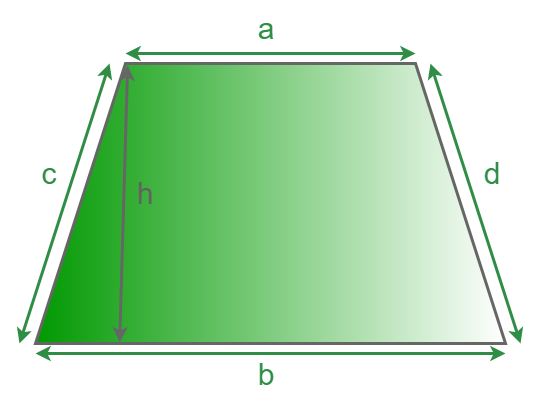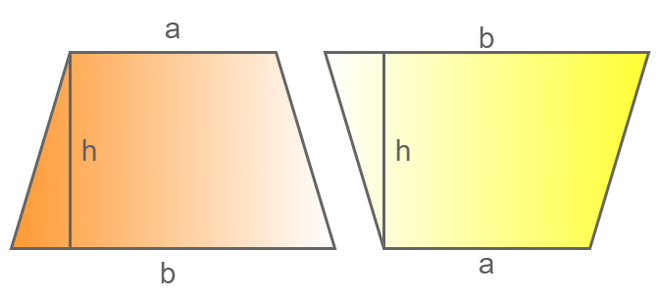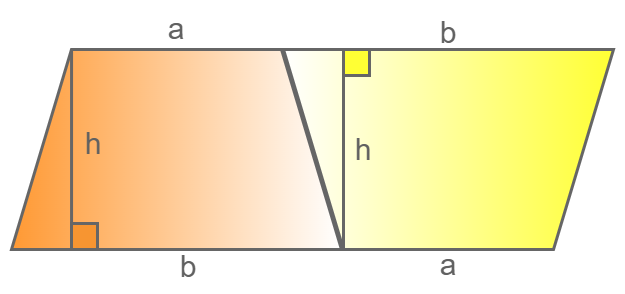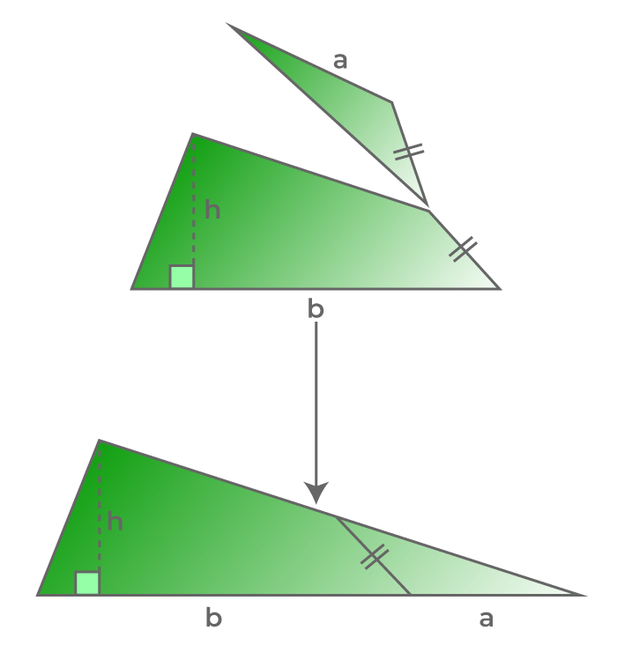GeeksforGeeks App
Open AppBrowser
Continue

# Area of Trapezium

Area of trapezium is the number of unit squares that can fit into the trapezium, and it is measured in square units. The trapezium, which is also known as the trapezoid, is a closed quadrilateral that contains a pair of parallel sides, whereas the other pair of sides are not parallel. The sides may or may not vary in length.

The parallel sides of the trapezium are called the bases of the trapezium. The distance between the bases is known as the height of the trapezium. The height of the trapezium is also known as the altitude. The non-parallel sides of the trapezium are known as the legs. The sum of the interior angles of the trapezium is 360°. It has 4 sides and 4 vertices.

## What is Area of Trapezium?

The area of the trapezium is defined as the region covered by the trapezium in 2-dimensional space. The area of trapezium is measured in square units, for example, meter2, centimeter2, inches2, etc. The area of the trapezium depends upon the height of the trapezium and the length of the parallel sides. Let’s understand in detail the formula for the area of a trapezium and how to derive it.## Area of Trapezium Formula

The area of any closed figure is the space enclosed within this object. The area of a trapezium or trapezoid is obtained using parallel lines and the distance between them. In the case of trapezium, the area is given by,

Area = 1/2 × (Sum of parallel sides) × (Distance between them)

Let’s assume the a and b to be the parallel sides of the trapezium and h to be the distance between them. Let the area of the trapezium be denoted by A. We have,

A = 1/2 × (a + b) × h square units

## How to Derive Area of Trapezium Formula?

The formula for the area of a trapezium can be obtained in two different ways,

### Derivation of Area of Trapezium Formula Using a Parallelogram

In order to find the area of a trapezium formula using a parallelogram, take two trapeziums that are the same (equal sides and angles), their parallel sides are a and b, and the height of the trapezium is h. Place the second trapezium upside down. It is clear that in this way if both the trapeziums are joined, it will become a parallelogram.Now, after joining both trapeziums, the trapeziums will form one parallelogram,Let’s say that the area of one trapezium is A, and the area of a parallelogram will be twice the area of the trapezium, that is, 2A. The area of a parallelogram is base × height. Therefore,

2A = Base × Height

Base = (a + b)

Height = h

2A = (a + b) × h

A = 1/2 × (a + b) × h

### Derivation of Area of Trapezium Formula Using a Parallelogram

Consider a trapezium with parallel sides as a and b and height as h. In order to find the area of the trapezium formula using a triangle, bisect the non-parallel side of the triangle, join it from the corner to make a small triangle, flip the triangle and make it into a bigger triangle (As shown below).It is observed that the area of the bigger triangle and the area of the original trapezium are equal. The base of the triangle is (a + b). The area of the triangle is given as,

A = 1/2 × base × height

A = 1/2 × (a + b) × h = Area of Trapezium

## Area of Isosceles Trapezium

The area of an isosceles trapezium or isosceles trapezoid is calculated by multiplying the height of the trapezium by the mean of the parallel sides. An isosceles trapezium is a trapezium with congruent base angles and congruent non-parallel sides. Suppose the parallel sides of the isosceles trapezium are “a” and “b” and the height of the trapezium is “h”, then the area of the isosceles trapezium is,

A = (a + b)/2 × h

## Area of Trapezium without Height

Area of the Trapezium can also be calculated even when the height is not given. This is done by following the steps discussed below:

Suppose a trapezium ABCD is given with sides as a, b, c, and d respectively, and its diameter is given as D.

Step 1: Divide the given trapezium into two triangles using the diameter as, ΔABD and ΔBCD

Step 2: Calculate the area of triangles ΔABD and ΔBCD separately by using Heron’s Formula as all the sides of both triangles are known.

Step 3: Add both the areas of triangles obtained in step 2.

This is the required area of the Trapezium and it is measured in square units.

## How to Calculate the Area of Trapezium?

The area of the trapezium can be found using the following sequence of steps:

Step 1: Calculate the lengths of the parallel sides (bases) of the trapezium.

Step 2: The sum of the bases of the trapezium is calculated.

Step 3: The value of the sum of bases is multiplied by the height or altitude of the trapezium and then by 1/2.

Step 4: The answer is then further simplified and is written in terms of square units.

## Perimeter of Trapezium

The perimeter of the trapezium is defined as the sum of all the sides of the trapezium. A trapezium has 2 parallel sides and two non-parallel sides. Therefore, the perimeter of the trapezium is the sum of parallel sides and the sum of nonparallel sides. The formula for the perimeter of a trapezium when the sides are “a”, “b”, “c”, and “d” is given by,

Perimeter (P) = (a + b + c + d) units

## Properties of Trapezium

The basic properties of a trapezium are similar to the properties of quadrilaterals with some specific properties to it. Below are the properties of trapezium,

• A trapezium is a two-dimensional figure.
• The bases of the trapezium are parallel to each other.
• The diagonals of an isosceles trapezium are equal in length, and they always intersect each other.
• The sum of the adjacent interior angles is 180°, and the sum of all the interior angles of a trapezium is 360°.

## Applications of Area of Trapezium

Area of Trapezium is a highly used formula that is frequently used in mathematics to find the area of various types of figures used in our daily life.

• It is important to find the area of various daily objects such as tables, land, etc.
• It is used in determining the area and plane of various objects.

## Solved Examples on the Area of Trapezium

Example 1: Find the area of the trapezium with the sum of the parallel sides being 40 m and the height being 20 m.

Solution:

Sum of the parallel sides = 40 m

Height of the trapezium = 20 m

As we know that, Area of trapezium = 1/2 × (Sum of the parallel sides) × Height

Area of trapezium = 1/2 × (40) × 20

Area of trapezium = 400 m2

Example 2: Find the sum of the parallel side of the trapezium if its area is 2500 cm2 and height is 50 cm.

Solution:

Here we have to find the sum pf parallel sides of the trapezium

Area of the trapezium = 2500 cm2

Height of the trapezium = 50 cm

As we know that, Area of trapezium = 1/2 × (Sum of the parallel sides) × Height

2500 = 1/2 × (Sum of the parallel sides) × 50

Sum of the parallel sides = (2500 × 2) / 50

Sum of the parallel sides = 100 cm

Example 3: Calculate the height of a trapezium if the sum of the parallel sides is 200 cm, and the area of the trapezium is 5000 cm2.

Solution:

Here we have to find the height of the trapezium

Area of the trapezium = 5000 cm2

Sum of the parallel sides of the trapezium = 200 cm

As we know that, Area of trapezium = 1/2 × (Sum of the parallel sides) × Height

5000 = 1/2 × 200 × Height

Height = (5000 × 2)/200

Height = 50 cm

Example 4: If the sum of the parallel sides of the trapezium is double the height and the area of the trapezium is 400 m2. Then find the sum of the parallel sides of the trapezium and its height.

Solution:

Area = 400 m2

Sum of the parallel sides of the trapezium is double its height

Let us assume, Height of the trapezium = x, than

Sum of the parallel sides of the trapezium = 2x

As we know that, Area of trapezium = 1/2 × (Sum of the parallel sides) × Height

400 = 1/2 × 2x × x

400 = 1/2 × 2x2

400 = x2

x = √400

x = 20 m

Thus,

Height of the trapezium = x = 20 m

Sum of the parallel sides of the trapezium = 2x = 2 × 20 = 40 m

## FAQs on Area of Trapezium

Question 1: What do you mean by the area of a Trapezium?

The area of trapezium is defined as the total space covered by the closed shape of the trapezium, it is calculated by multiplying the height of the trapezium to the sum of its parallel sides.

Question 2: How do you calculate the area of a trapezium if only the base and height are given?

The area of trapezium can be calculated by multiplying the average of the base lengths with the height of the trapezium.

Question 3: How do you calculate the area of a trapezium when the height of the trapezium is not given?

In case, the height of the trapezium is not provided and the sides of the trapezium is given, divide the trapezium into two triangles and separately find their areas. Once, the area of triangles are obtained, sum them to obtain the area of trapezium.

Question 4: What is a Trapezium?

A trapezium is a 2-D quadrilateral with a pair of non-parallel sides and a pair of parallel sides. The sum of all interior angles of a trapezium is equal to 360 degrees.

Question 5: What are the Formulas for the Perimeter and the Area of the Trapezium?

The perimeter and the area of a trapezium can be calculated using the formulas:

A = ½ × (a + b) × h
P = a + b + c + d

where,
a, b, c, and d are the length of the sides of a trapezium
h is the height of the trapezium

### Related Resources

My Personal Notes arrow_drop_up
Related Tutorials